# Machine Learning & Big Data @Andy Sloane
@a1k0n
http://a1k0n.net

Jan 27, 2015

## What do we need ML for?

• Recommendations
• Related Artists

# Recommendations

### The Discover page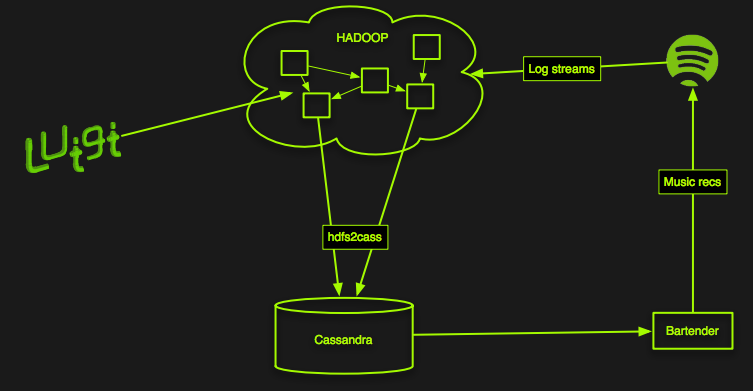Okay, but how do we come up with recommendations?
Collaborative filtering!

### Collaborative filtering

Great, but how does that actually work?
• Each time a user plays something, add it to a matrix
• Compute similarity, somehow, between items based on who played what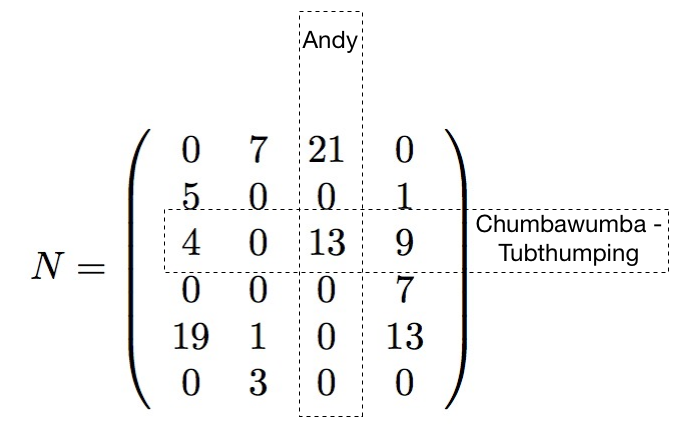### Collaborative filtering

• So compute some distance between every pair of rows and columns
• That's just O($\frac{{60M}^2}{2}$) = O($1.8\times 10^{15}$) operations... O_O
• We need a better way...

(BTW: Twitter has a decent approximation that can actually make this work, called DIMSUM:
I've tried it but don't have results to report here yet :(

### Collaborative filtering

#### Latent factor models

Instead, we use a "small" representation for each user & item: $f$-dimensional vectors(here, $f=2$)

and approximate the big matrix with it.

## Why vectors?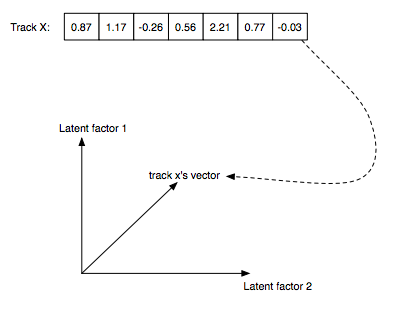• Very compact representation of musical style or user's taste
• Only like 40-200 elements (2 shown above for illustration)

### Recommendations via dot products### Another example of tracks in two dimensions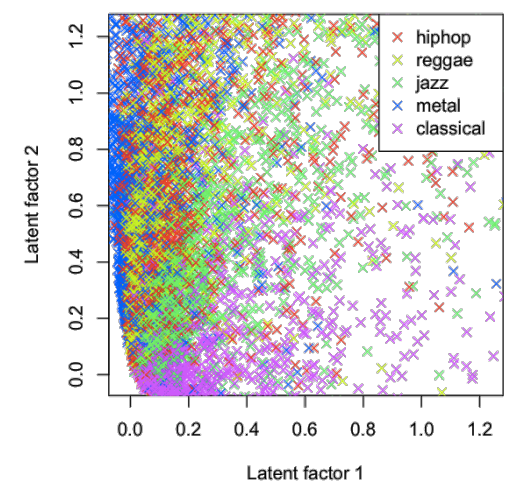## Implicit Matrix Factorization

Hu, Koren, Volinsky - Collaborative Filtering for Implicit Feedback Datasets

Tries to predict whether user $u$ listens to item $i$:

$P = \left( \begin{array}{cccc} 0 & 0 & 0 & 1 \\ 0 & 1 & 1 & 0 \\ 0 & 0 & 1 & 0 \\ 1 & 0 & 0 & 1 \end{array} \right) \approx \left( \begin{array}{ccc} & X & \end{array} \right) \left( \begin{array}{c} \\ Y^T \\ \\ \end{array} \right)$

$Y$ is all item vectors, $X$ is all user vectors

"implicit" because users don't tell us what they like, we only observe what they do/don't listen to

## Implicit Matrix Factorization

Goal: make $x_u \cdot y_i$ close to 1 for things each user has listened to, 0 for everything else.
• $x_u$ — user $u$'s vector
• $y_i$ — item $i$'s vector
• $p_{ui}$ — 1 if user $u$ played item $i$, 0 otherwise
• $c_{ui}$ — "confidence", ad-hoc weight based on number of times user $u$ played item $i$; e.g., $1 + \alpha \cdot \tt{plays}_{ui}$
• $\lambda$ — regularization penalty to avoid overfitting
Minimize: $\sum_{u,i} c_{ui} \left( p_{ui} - x_{u}^{T} y_{i} \right)^2 + \lambda \left(\sum_u ||x_u||^2 + \sum_i ||y_i||^2 \right)$

## Alternating Least Squares

Solution: alternate solving for all users $x_u$: $x_u = (Y^T Y + Y^T (C^u - I) Y + \lambda I)^{-1} Y^T C^u p_{u\cdot}$ and all items $y_i$: $y_i = (X^T X + X^T (C^i - I) X + \lambda I)^{-1} X^T C^i p_{\cdot i}$
• $Y^T Y$ = $f$ x $f$ matrix, sum of outer products of all items
• $Y^T (C^u - I) Y$ same, except only items the user played
• $Y^T C^u p_u$ = weighted $f$-dimensional sum of items the user played

## Alternating Least Squares

Key point: each iteration is linear in size of input, even though we are solving for all users x all items, and needs only $f^2$ memory to solve

No learning rates, just a few tunable parameters ($f$, $\lambda$, $\alpha$)

All you do is add stuff up, solve an $f$x$f$ matrix problem, and repeat!

We use $f = 40$ dimensional vectors for recommendations

Matrix/vector math using numpy in Python, breeze in scala

## Alternating Least Squares

### Adding lots of stuff up

• Problem: any user (60M) can play any item (4M)
• thus we may need to add any user's vector to any item's vector
• If we put user vectors in memory, it takes a lot of RAM!
• Worst case: 60M users * 40 dimensions * sizeof(float) = 9.6GB of user vectors
• ...too big to fit in a mapper slot on our cluster

### Adding lots of stuff up

Solution: Split the data into a matrix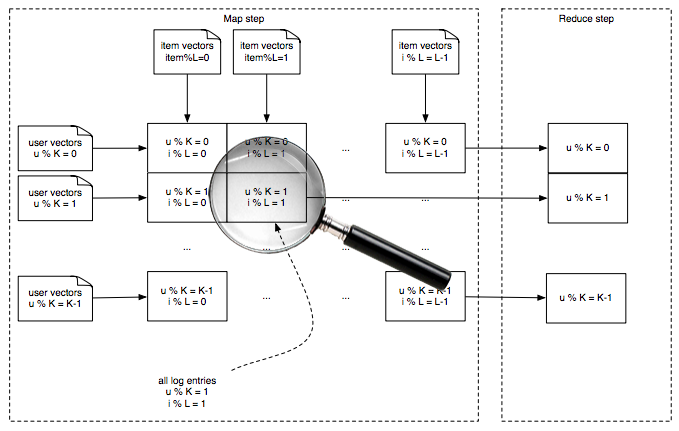Most recent run made a 14 x 112 grid

## One map shard

Input is a bunch of (user, item, count) tuples
user is the same modulo K for all users
item is the same modulo L for all items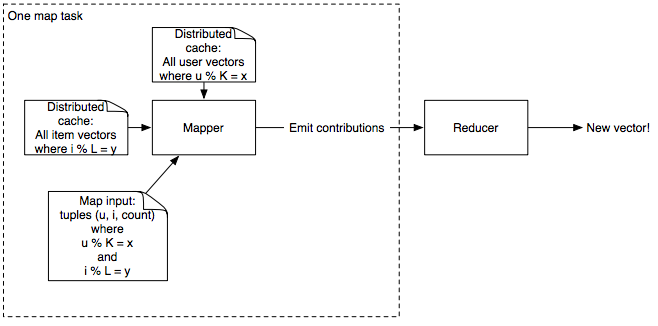e.g., if K = 4, mapper #1 gets users 1, 5, 9, 13, ...

Add up vectors from every (user, item, count) data point

def mapper(self, input):  # Luigi-style python job
user, item, count = parse(input)
conf = AdHocConfidenceFunction(count)   # e.g. 1 + alpha*count
# add up user vectors from previous iteration
term1 = conf * self.user_vectors[user]
term2 = np.outer(user_vectors[user], user_vectors[user])
* (conf - 1)
yield item, np.array([term1, term2])

def reducer(self, item, terms):
term1, term2 = sum(terms)
item_vector = np.solve(
self.YTY + term2 + self.l2penalty * np.identity(self.dim),
term1)
yield item, item_vector

Then flip users ↔ items and repeat!

## Finding Recommendations

60M users x 4M recommendable items
• For each user, how do we find the best items given their vector?
• Brute force is O(60M x 4M x 40) = O(9 peta-operations)!
• Instead, use an approximation based on locality sensitive hashing (LSH)

## Locality-Sensitive Hashing

### Annoy - github.com/spotify/annoy

• Pre-built read-only database of item vectors
• Internally, recursively splits random hyperplanes
• Nearby points likely on the same side of random split
• Builds several random trees (a forest) for better approximation
• Given an $f$-dimensional query vector, finds similar items in database
• Index loads via mmap, so all processes on the same machine share RAM
• Queries are very, very fast, but approximate
• Python implementation available, Java forthcoming

### Generating recommendations

• Annoy index for all items is only 1.2GB
• I have one on my laptop... Live demo!
• Could serve up nearest neighbors at load time, but we precompute Discover on Hadoop

### Generating recommendations in parallel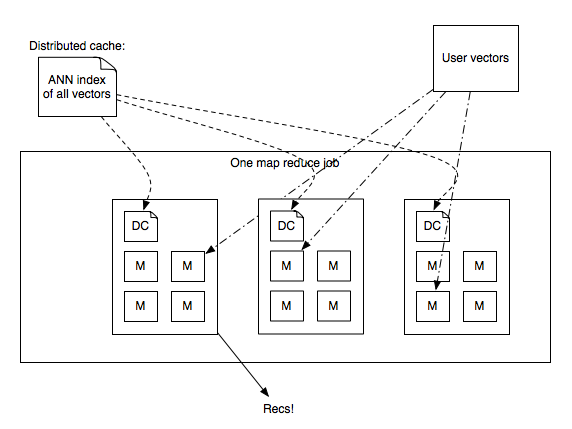• Send annoy index in distributed cache, load it via mmap in map-reduce process
• Reducer loads vectors + user stats, looks up ANN, generates recommendations.

# Related Artists

## Related Artists

• Great for music discovery
• Essential for finding believable reasons for latent factor-based recommendations• When generating recommendations, run through a list of related artists to find potential reasons

### Similar items use cosine distance

• Cosine is similar to dot product; just add a normalization step
• Helps "factor out" popularity from similarity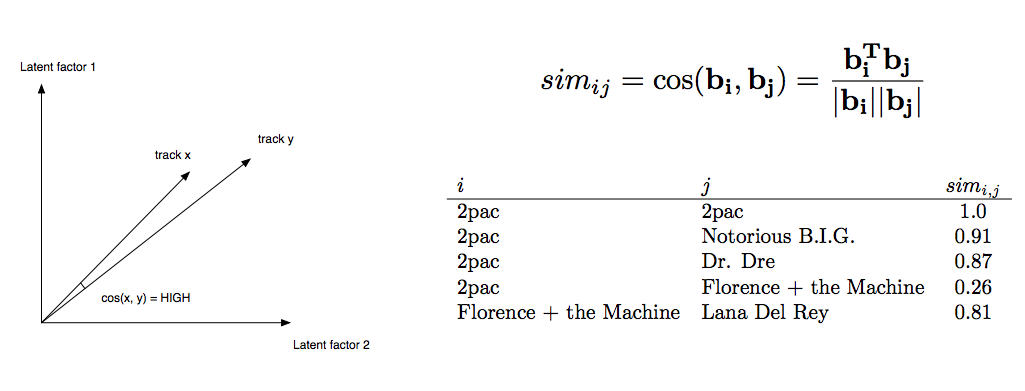## Related Artists

### How we build it

• Similar to user recommendations, but with more models, not necessarily collaborative filtering based
• Implicit Matrix Factorization (shown previously)
• "Vector-Exp", similar model but probabilistic in nature, trained with gradient descent
• Echo Nest "cultural similarity" — based on scraping web pages about music!
• Query ANNs to generate candidates
• Score candidates from all models, combine and rank
• Pre-build table of 20 nearest artists to each artist

ML-wise, exactly the same as Related Artists!
• For each track, generate candidates with ANN from each model
• Score w/ all models, rank with ensemble
• Store top 250 nearest neighbors in a database (Cassandra)
• Thumbs up → load more tracks from the thumbed-up song
• Thumbs down → remove that song / re-weight tracks

# Upcoming work

### Deep learning based item similarity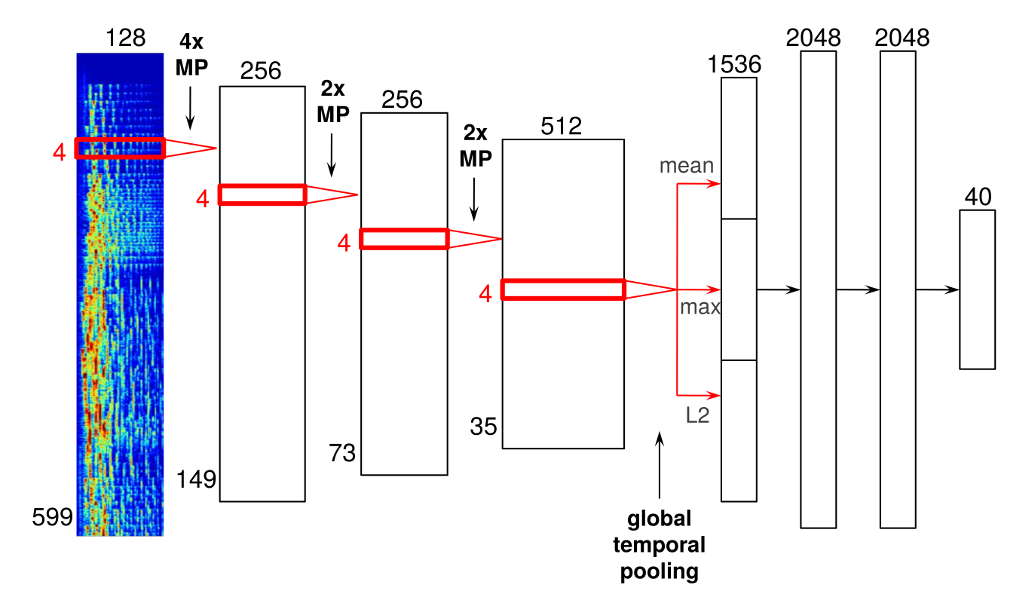http://benanne.github.io/2014/08/05/spotify-cnns.html

# Upcoming work### Audio fingerprint based content deduplication

• ~1500 Echo Nest Musical Fingerprints per track
• Min-Hash based matching to accelerate all-pairs similarity
• Fast connected components using Hash-to-Min algorithm - $O(\log d)$ mapreduce steps
http://arxiv.org/pdf/1203.5387.pdf

# Thanks!

I can be reached here:

Andy Sloane
Email: andy@a1k0n.net